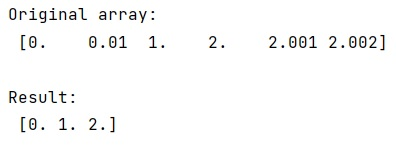# Python - How to filter integers in NumPy float array?

Given float NumPy array. We have to filter integers in NumPy float array in Python. By Pranit Sharma Last updated : April 08, 2023

Suppose that we are given a float array that contains some integer numbers and we need to filter out the integer numbers from this float array.

For example, if we are given an array as: [[0.0, 0.01, 1.0, 2.0, 2.001, 2.002]] from which we need to filter out 1.0 and 2.0 as integers.

## Filtering integers in NumPy float array

To filter integers in the NumPy float array, we can mask the array with whether each element is equal to it as an integer or not.

Let us understand with the help of an example,

## Python code to filter integers in NumPy float array

```# Import numpy
import numpy as np

# Creating an array
arr = np.array([0.0, 0.01, 1.0, 2.0, 2.001, 2.002])

# Display array
print("Original array:\n",arr,"\n")

# Filtering out integer values
res = arr[arr == arr.astype(int)]

# Display result
print("Result:\n",res,"\n")
```

### Output